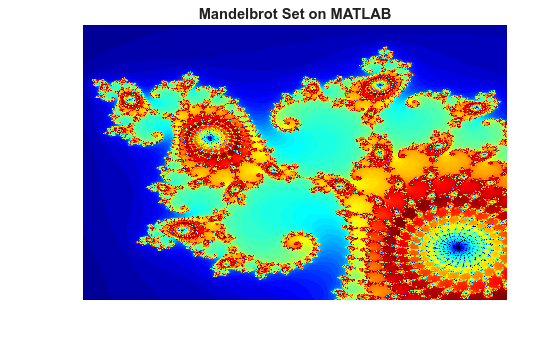# GPU Code Generation: The Mandelbrot Set

This example shows how to generate CUDA® code from a simple MATLAB® function by using GPU Coder™. A Mandelbrot set implementation by using standard MATLAB commands acts as the entry-point function. This example uses the `codegen` command to generate a MEX function that runs on the GPU. You can run the MEX function to check for run-time errors.

### Third-Party Prerequisites

#### Required

This example generates CUDA MEX and has the following third-party requirements.

• CUDA enabled NVIDIA® GPU and compatible driver.

#### Optional

For non-MEX builds such as static, dynamic libraries or executables, this example has the following additional requirements.

### Verify GPU Environment

To verify that the compilers and libraries necessary for running this example are set up correctly, use the `coder.checkGpuInstall` function.

```envCfg = coder.gpuEnvConfig('host'); envCfg.BasicCodegen = 1; envCfg.Quiet = 1; coder.checkGpuInstall(envCfg);```

### Mandelbrot Set

The Mandelbrot set is the region in the complex plane consisting of the values ${z}_{0}$ for which the trajectories defined by

`${z}_{k+1}={{z}_{k}}^{2}+{z}_{0},k=0,1,...$`

remain bounded at $k\to \infty$. The overall geometry of the Mandelbrot set is shown in the figure. This view does not have the resolution to show the richly detailed structure of the fringe just outside the boundary of the set.

### Define Input Regions

Pick a set of limits that specify a highly zoomed part of the Mandelbrot set in the valley between the main cardioid and the $p/q$ bulb to its left. A `1000x1000` grid of $Re\left\{x\right\}$ and $Im\left\{y\right\}$ is created between these two limits. The Mandelbrot algorithm is then iterated at each grid location. An iteration number of 500 is enough to render the image in full resolution.

```maxIterations = 500; gridSize = 1000; xlim = [-0.748766713922161, -0.748766707771757]; ylim = [ 0.123640844894862, 0.123640851045266]; x = linspace( xlim(1), xlim(2), gridSize ); y = linspace( ylim(1), ylim(2), gridSize ); [xGrid,yGrid] = meshgrid( x, y );```

### The Mandelbrot Entry-Point Function

The `mandelbrot``_c``ount.m` entry-point function contains a vectorized implementation of the Mandelbrot set based on the code provided in the e-book Experiments with MATLAB by Cleve Moler. The `%#codegen` directive turns on MATLAB for code generation error checking. When GPU Coder encounters the `coder.gpu.kernelfun` pragma, it attempts to parallelize all the computation within this function, and then maps it to the GPU.

`type mandelbrot_count`
```function count = mandelbrot_count(maxIterations, xGrid, yGrid) %#codegen % Copyright 2016-2019 The MathWorks, Inc. z0 = xGrid + 1i*yGrid; count = ones(size(z0)); % Map computation to GPU. coder.gpu.kernelfun; z = z0; for n = 0:maxIterations z = z.*z + z0; inside = abs(z)<=2; count = count + inside; end count = log(count); ```

### Test the Functionality of `mandelbrot``_c``ount`

Run the `mandelbrot``_c``ount` function with the xGrid, yGrid values that were previously generated, and then plot the results.

```count = mandelbrot_count(maxIterations, xGrid, yGrid); figure(2), imagesc( x, y, count ); colormap( [jet();flipud( jet() );0 0 0] ); title('Mandelbrot Set on MATLAB'); axis off```### Generate CUDA MEX for the Function

To generate CUDA MEX for the `mandelbrot``_c``ount` function, create a GPU code configuration object and run the `codegen` command. Because of architectural differences between the CPU and GPU, numeric verification does not always match. This scenario is true when using the single data type in your MATLAB code and performing accumulation operations on these single data type values. Like this Mandelbrot example even the double data types cause numeric errors. One reason for this mismatch is that the GPU floating-point units use fused Floating-point Multiply-Add (FMAD) instructions and the CPU does not use these instructions. The `fmad=false` option that is passed to the `nvcc` compiler turns off this FMAD optimization.

```cfg = coder.gpuConfig('mex'); cfg.GpuConfig.CompilerFlags = '--fmad=false'; codegen -config cfg -args {maxIterations,xGrid,yGrid} mandelbrot_count```
```Code generation successful: View report ```

### Run the MEX Function

After generating a MEX function, verify that it has the same functionality as the original MATLAB entry-point function. Run the generated `mandelbrot``_c``ount_mex` and plot the results.

```countGPU = mandelbrot_count_mex(maxIterations, xGrid, yGrid); figure(2), imagesc( x, y, countGPU ); colormap( [jet();flipud( jet() );0 0 0] ); title('Mandelbrot Set on GPU'); axis off```